Question

# Determine the internal normal force, shear force

Determine the internal normal force, shear force, and moment at points C and D in the simply supported beam using method of sections. Point D is A located just to the left of the 5-kN force. Also, draw shear and moment diagrams using the graphical method.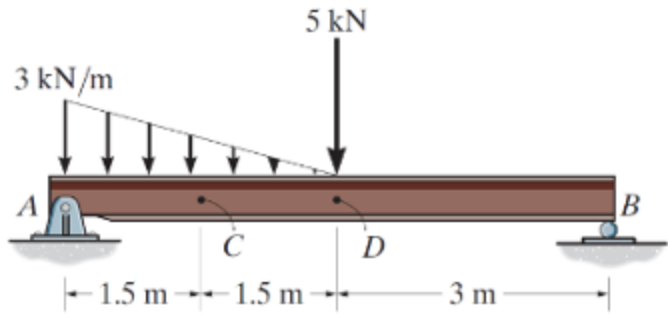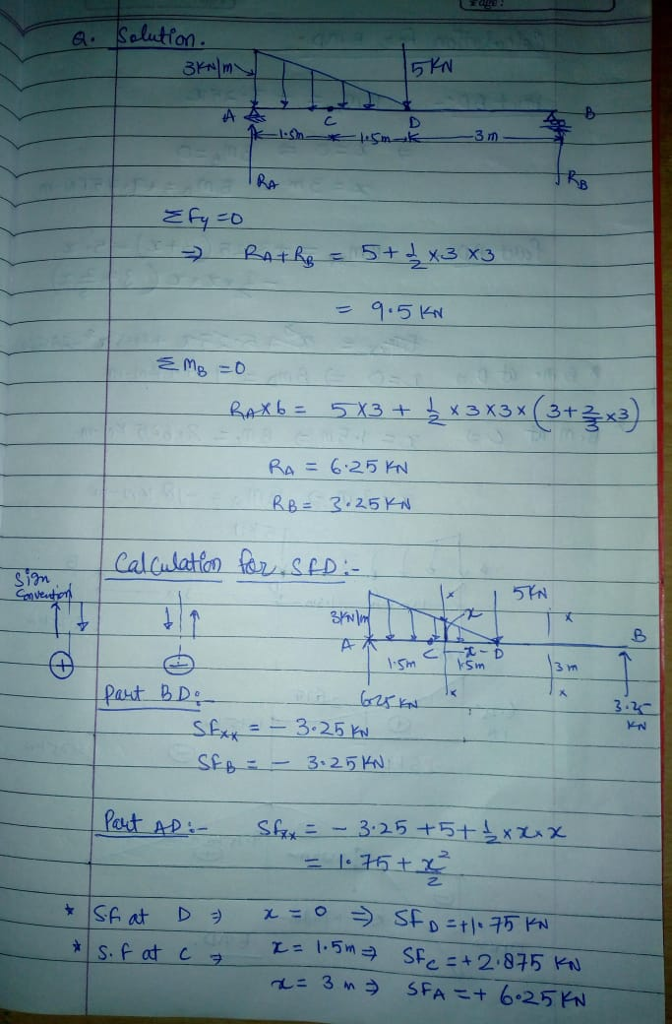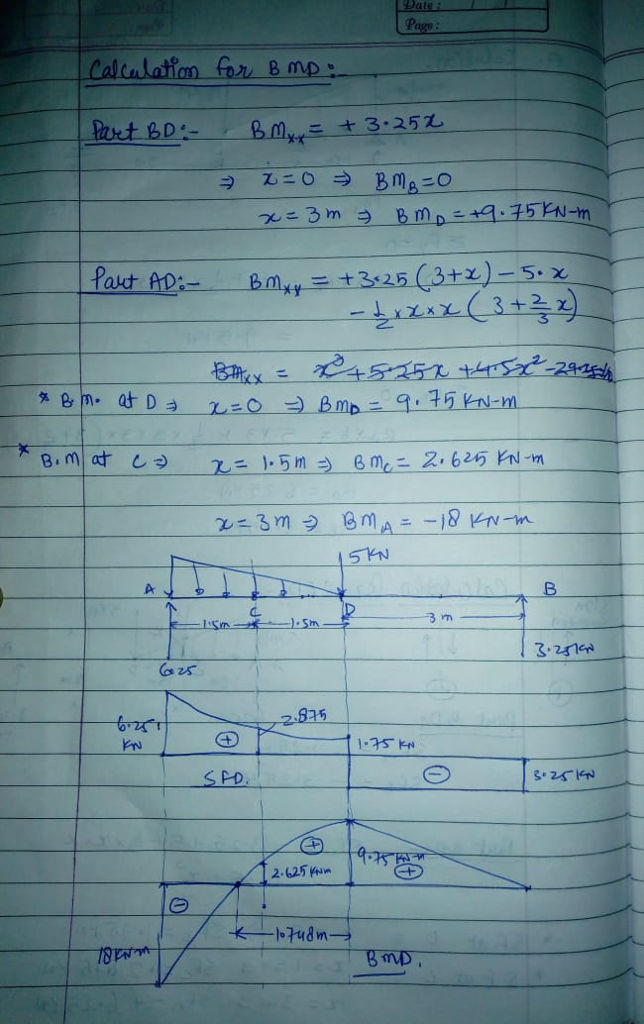#### Earn Coins

Coins can be redeemed for fabulous gifts.

Similar Homework Help Questions
• ### Determine the internal normal force, shear force, and moment at points C and D in the...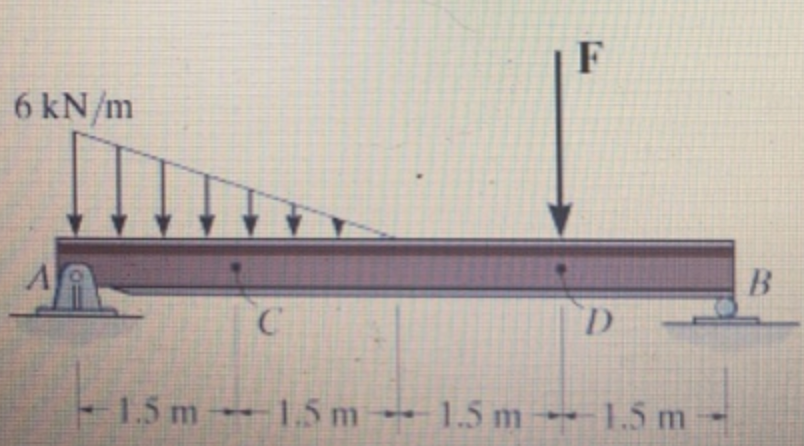Determine the internal normal force, shear force, and moment at points C and D in the simply supported beam. Point D is located just to the left of the F = 15 kN concentrated load. (Figure 1)

• ### Determine the internal normal force, shear force, and moment at points and D in the simply supported beam.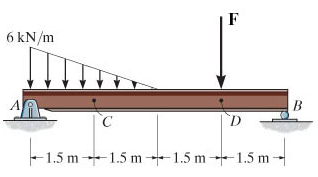Determine the internal normal force, shear force, and moment at points and D in the simply supported beam. Point Dis located just to the left of the F = 14 kN concentrated load.(Figure 1)

• ### 2) A beam supports the loading shown below. Determine the internal normal force, shear force and...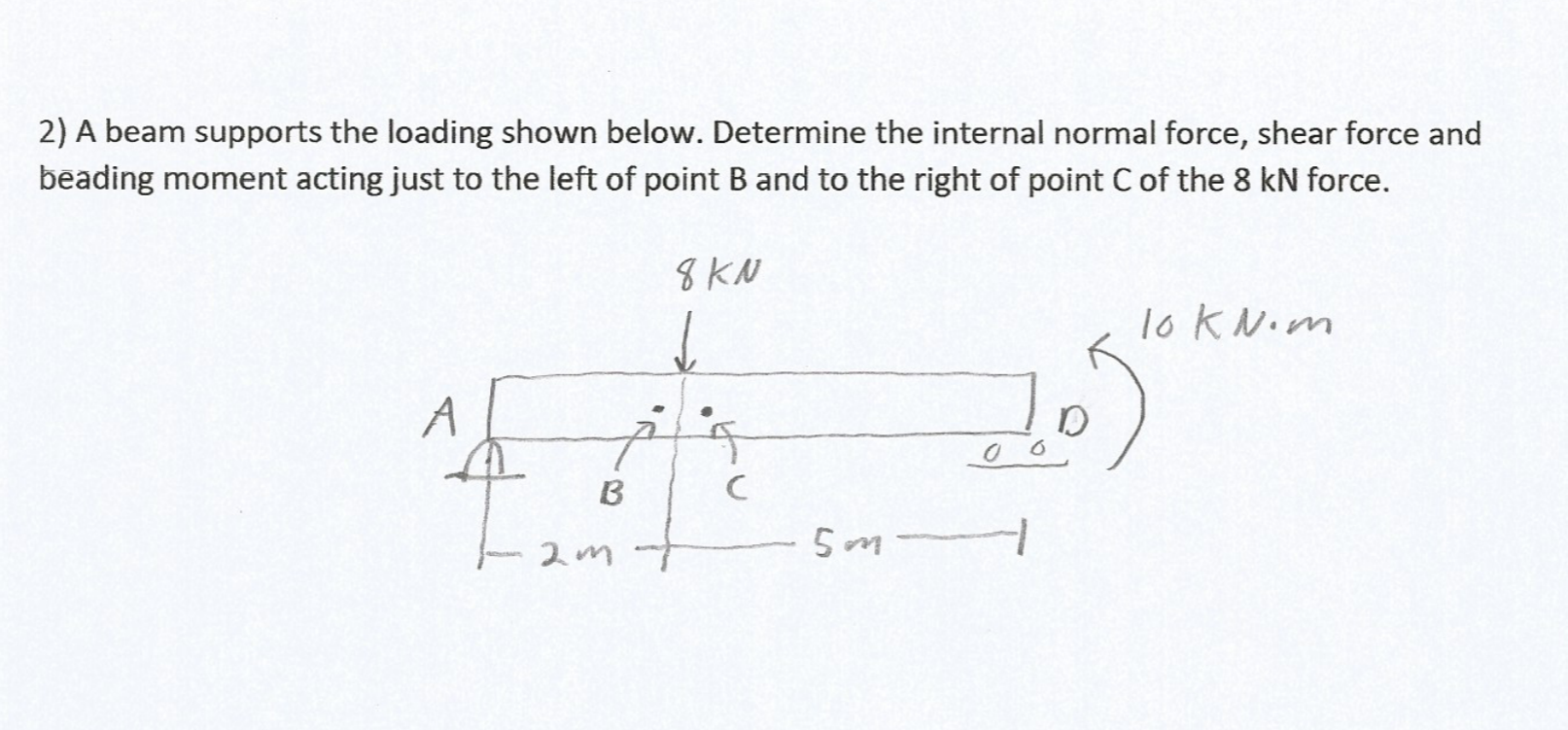2) A beam supports the loading shown below. Determine the internal normal force, shear force and beading moment acting just to the left of point B and to the right of point C of the 8 kN force. 8 KN loknom A D oo 6 ) B C 2m 5m 1

• ### 2) A beam supports the loading shown below. Determine the internal normal force, shear force and...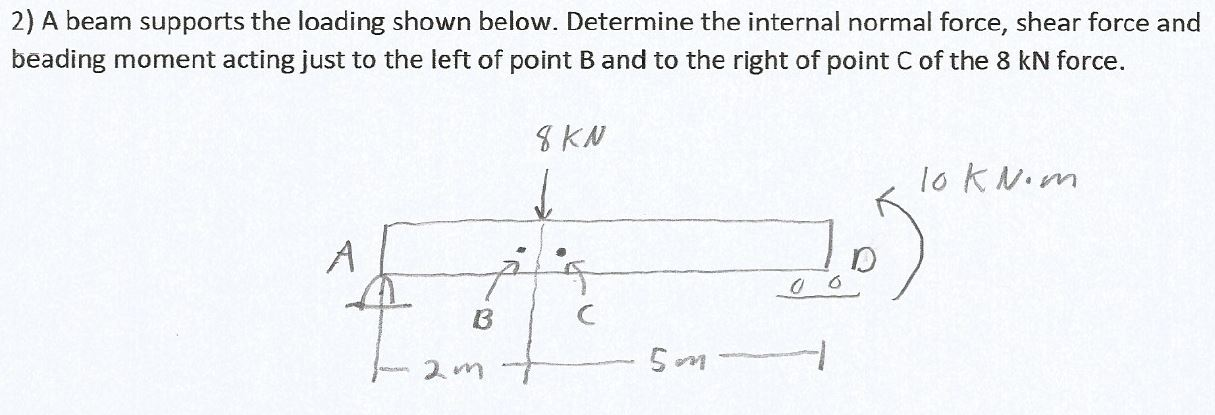2) A beam supports the loading shown below. Determine the internal normal force, shear force and beading moment acting just to the left of point B and to the right of point Cof the 8 kN force. 8 KN loknom A D t 0 0 B C 5m

• ### Determine the internal force shear force and moment at point c. ENGR 2301 Statics Spring 2014 Name Exam 3 Sbow all w...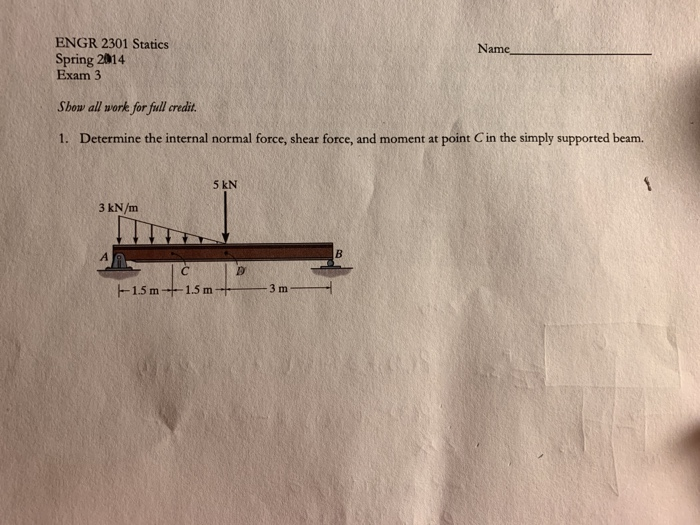Determine the internal force shear force and moment at point c. ENGR 2301 Statics Spring 2014 Name Exam 3 Sbow all work for full credit. 1. Determine the internal normal force, shear force, and moment at point C in the simply supported beam. 5 kN 3 kN/m --15 m -15 m3m

• ### The number of significant digits is set to 3 A positive internal shear force V •...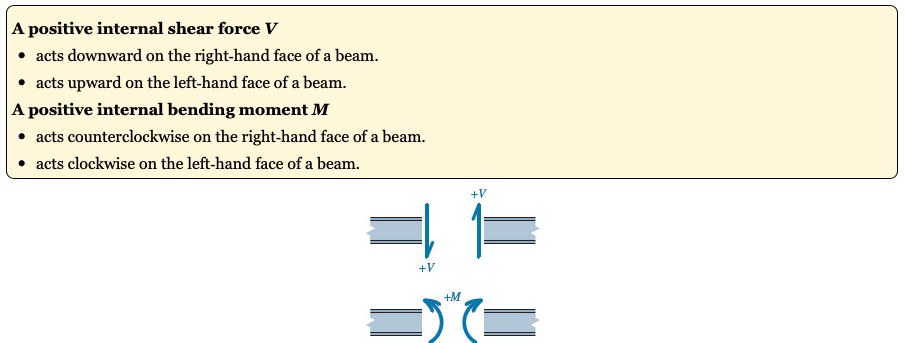The number of significant digits is set to 3 A positive internal shear force V • acts downward on the right-hand face of a beam. • acts upward on the left-hand face of a beam. A positive internal bending moment M • acts counterclockwise on the right-hand face of a beam. • acts clockwise on the left-hand face of a beam. = = Use the graphical method to construct the shear-force and bending-moment diagrams for the beam shown. Label all...

• ### Question 5 10 points Save Answ Draw the shear-force and bending-moment diagrams for the simply supported...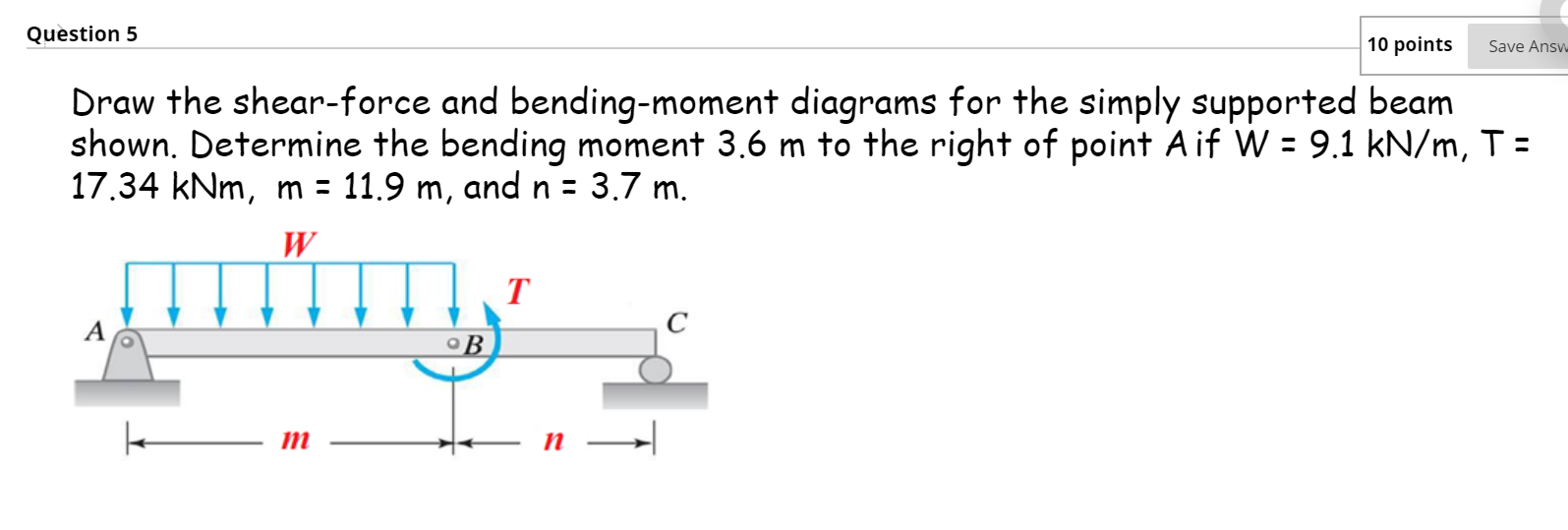Question 5 10 points Save Answ Draw the shear-force and bending-moment diagrams for the simply supported beam shown. Determine the bending moment 3.6 m to the right of point A if W = 9.1 kN/m, T = 17.34 kNm, m = 11.9 m, and n = 3.7 m. W T С о В n Question 6 10 points Save Answer Draw the shear-force and bending-moment diagrams for the simply supported beam shown. Determine the shear force 3.3 m to the...

• ### Use the graphical method to construct the shear-force and bending-moment diagrams for the beam shown.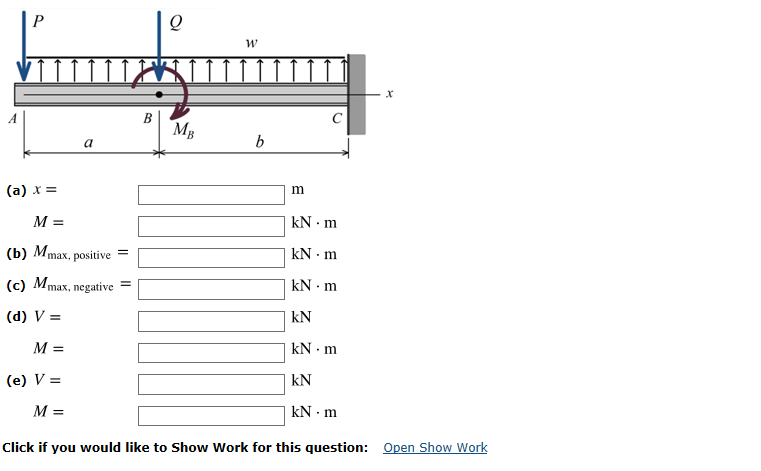Use the graphical method to construct the shear-force and bending-moment diagrams for the beam shown. Label all significant points on each diagram and identify the maximum moments along with their respective locations. For all answers entered, use the sign convention for shear forces and bending moments. (a) Find the location x and the corresponding bending moment M at the one point between A and B at which the shear force equals zero. (b) Consider the entire beam and determine the maximum positive...

• ### Use the graphical method to construct the shear-force and bending-moment diagrams for the beam shown. Label...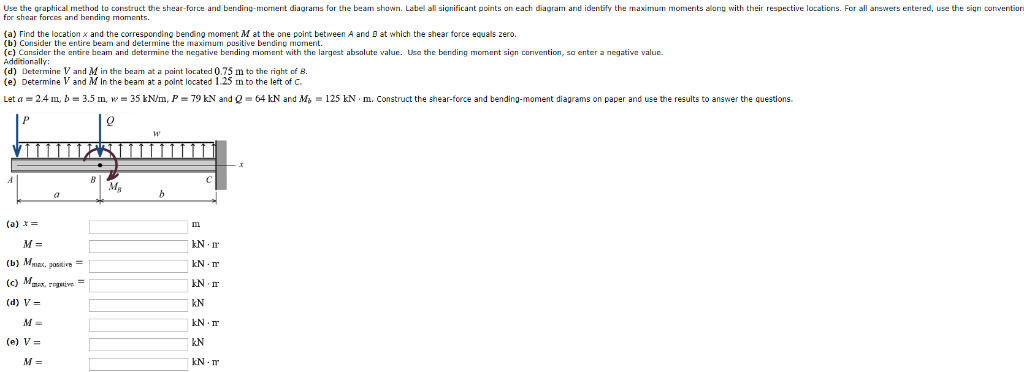Use the graphical method to construct the shear-force and bending-moment diagrams for the beam shown. Label all significant points on each diagram and identify the maximum moments along with their respective locations. For all answers entered, use the sign convention for shear forces and bending moments (a) Find the location x and the corresponding bending moment M at the one point between A and D at which the shear force equals zero. (b) Consider the entire beam and determine the...

• ### Draw the shear force and bending-moment diagrams for the simply supported beam shown. Label each diagram...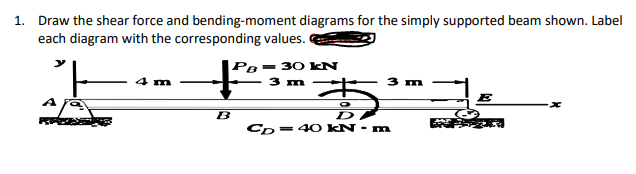Draw the shear force and bending-moment diagrams for the simply supported beam shown. Label each diagram with the corresponding values 1. Draw the shear force and bending-moment diagrams for the simply supported beam shown. Label each diagram with the corresponding values. 3 Pe= 30 KN 4 m - m 3 m - C -40 kN - m